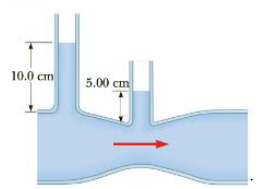Chapter 9, Problem 45P

Chapter
Section
Textbook Problem

The inside diameters of the larger portions of the horizontal pipe depicted in Figure P9.45 are 2.50 era. Water flows to the right at a rate of 1.80 × 10−4 m3/s. Determine the inside diameter of the constriction.Figure P9.45

To determine
The inside diameter of the constriction.

Explanation

Section1:

To determine: The flow speed of water inside the larger section.

Answer: The flow speed of water inside the larger section is 0.367m/s .

Explanation: The flow speed of water inside of the larger section is calculated using continuity equation as follows. A1v1=flowratev1=flowrate/A1=4(flowrate)/πd12 .

Given info: The flow speed of water is 1.80×104m3/s and the inside diameter of the larger section of the pipe is 2.50cm .

The formula for the flow speed of water inside the larger section of the water is,

v1=4(flowrate)πd12 .

• d1 is inside diameter of the larger section.

Substitute 1.80×104m3/s for flowrate and 2.50cm for d1 to find v1 .

v1=4(1.80×104m3/s)(3.14)[(2.50cm)(0.01m1cm)]2=0.367m/s

Thus, the flow speed of water inside the larger section is 0.367m/s .

Section2:

To determine: The cross sectional area of the constriction.

Answer: The cross sectional area of the constriction is 1.70×104m2 .

Explanation: We use Bernoulli’s equation for the flow speed of water in the region of that constriction such that v22=v12+(2/ρ)(P1P2) by assuming that y1=y2 . The pressure difference between these two regions would be P1P2=Patm+ρgh1Patm+ρgh2=ρg(h1h2) which going to be substituted in the expression of the flow speed of the water in constriction region is v2=v12+2g(h1h2) .

Now we arrive that the area of constriction is A2=flowrate/v2=flowrate/(v12+2g(h1h2)) .

Given info: The flow speed of water in the larger section of the pipe is 0.367m/s , acceleration due to gravity is 9.80m/s2 , the water level in the left most stand pipe is 10

Still sussing out bartleby?

Check out a sample textbook solution.

See a sample solution

The Solution to Your Study Problems

Bartleby provides explanations to thousands of textbook problems written by our experts, many with advanced degrees!

Get Started